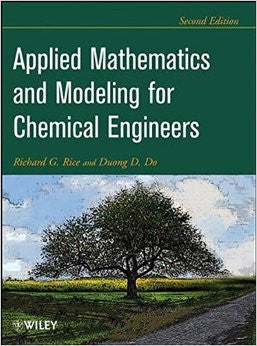# Applied Mathematics And Modeling For Chemical Engineers

: Brown Technical Book Store

: 9781118024720

: Book

\$99.95 \$133.00

#### Details

This Second Edition of the go-to reference combines the classical analysis and modern applications of applied mathematics for chemical engineers. The book introduces traditional techniques for solving ordinary differential equations (ODEs), adding new material on approximate solution methods such as perturbation techniques and elementary numerical solutions. It also includes analytical methods to deal with important classes of finite-difference equations. The last half discusses numerical solution techniques and partial differential equations (PDEs). The reader will then be equipped to apply mathematics in the formulation of problems in chemical engineering. Like the first edition, there are many examples provided as homework and worked examples.